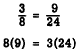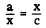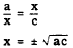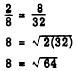Custom SearchOPERATIONS OF PROPORTIONS It is often advantageous to change the form of a proportion. There are rules for changing or combining the terms of a proportion without altering the equality between the members, These rules are simplifications of fundamental rules for equations; they are not new, but are simply adaptations of laws or equations presented earlier in this course. Rule 1. In any proportion, the product of the means equals the product of the extremes, This is perhaps the most commonly used rule of proportions. It provides a simple way to rearrange a proportion so that no fractions are present. In algebraic language the rule is illustrated as follows:To prove this rule, we note that the LCD of the two ratios a/b and c/d is bd. Multiplying both members of the equation in its original form by this LCD, we haveThe following numerical example illustrates the simplicity of rule 1:If one of the terms of a proportion is a variable to the first power as in         7:5 = x:6 the proportion is really a linear equation in one variable. Such an equation can be solved for the unknown. Equating the products of the means and extremes produces the following:Mean Proportional When the two means of a proportion are the same quantity, that quantity is called the MEAN PROPORTIONAL between the other two terms. In the proportionx is the mean proportional between a and c. Rule 2. The mean proportional between two quantities is the square root of their product. This rule is stated algebraically as follows:To prove rule 2, we restate the proportion and apply rule 1, as follows:Rule 2 is illustrated by the following numerical example:Integrated Publishing, Inc. - A (SDVOSB) Service Disabled Veteran Owned Small Business